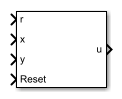# State-Feedback Controller

Discrete-time state-feedback controller with integral action

• Library:
• Simscape / Electrical / Control / General Control

•## Description

The State-Feedback Controller block implements a discrete-time state-feedback controller with integral action. Use this block to control linear systems with single or multiple inputs and single or multiple outputs. The integral action serves to eliminate steady-state error in the controlled outputs. You can define the controller using a precomputed optimal gain or use the state-space model of your system to generate this gain using pole placement.### Equations

The integral of the tracking error, xi, is an additional state that ensures zero steady-state error for the closed-loop system. The extended state vector is

`${x}_{e}=\left[\begin{array}{c}x\\ {x}_{i}\end{array}\right],$`

Where:

• x is the state vector.

• xi is the integral of the tracking error.

• xe is the extended state vector.

Therefore, the control action is

`$u=K{x}_{e},$`

Where:

• K is the feedback matrix, that is, the pole placement.

• u is the controller output.

### Assumptions

System state measurement and estimation occur outside the controller.

## Ports

### Input

expand all

Plant system reference signal.

Data Types: `single` | `double`

Measured or estimated system state vector.

Data Types: `single` | `double`

External reset signal (rising edge) for the integrator.

Data Types: `Boolean`

Plant system output signal.

Data Types: `single` | `double`

### Output

expand all

Control system output signal.

Data Types: `single` | `double`

## Parameters

expand all

Select the strategy for parameterizing controller gain:

• `State-feedback gain` — Specify the controller gain directly

• `Desired eigenvalues` — Specify the plant model and desired eigenvalues from which to generate the controller gain

Select the strategy for parameterizing the state-space matrices and desired poles for the controller. The block implementation is discrete regardless of this parameterization.

#### Dependencies

To enable this parameter, set State-feedback design to ```Desired eigenvalues```.

Controller feedback matrix. To determine the controller matrix, if you have a license for Control System Toolbox™, use the `lqr` or `lqi` function.

#### Dependencies

To enable this parameter, set State-feedback design to ```State-feedback gain```.

State matrix of the discrete-time state-space model. The A matrix must be square, with the number of rows and columns equal to the order of the system.

#### Dependencies

To enable this parameter, set State-feedback parameterization to `Discrete-time`.

Input matrix of the discrete-time state-space model. The B matrix must have the number of rows equal to the order of the system, and the number of columns equal to the number of system inputs.

#### Dependencies

To enable this parameter, set State-feedback parameterization to `Discrete-time`.

Output matrix of the discrete-time state-space model. The C matrix must have the number of rows equal the number of outputs of the system, and the number of columns equal to the order of the system.

#### Dependencies

To enable this parameter, set State-feedback parameterization to `Discrete-time`.

Feedthrough matrix of the discrete-time state-space model. The D matrix must have the number of rows equal to the number of system outputs, and the number of columns equal to the number of system inputs.

#### Dependencies

To enable this parameter, set State-feedback parameterization to `Discrete-time`.

State matrix of the continuous-time state-space model. The A matrix must be square, with the number of rows and columns equal to the order of the system.

#### Dependencies

To enable this parameter, set State-feedback parameterization to `Continuous-time`.

Input matrix of the continuous-time state-space model. The B matrix must have the number of rows equal to the order of the system, and the number of columns equal to the number of system inputs.

#### Dependencies

To enable this parameter, set State-feedback parameterization to `Continuous-time`.

Output matrix of the continuous-time state-space model. The C matrix must have the number of rows equal the number of outputs of the system, and the number of columns equal to the order of the system.

#### Dependencies

To enable this parameter, set State-feedback parameterization to `Continuous-time`.

Feedthrough matrix of the continuous-time state-space model. The D matrix must have the number of rows equal to the number of system outputs, and the number of columns equal to the number of system inputs.

#### Dependencies

To enable this parameter, set State-feedback parameterization to `Continuous-time`.

Value used to discretize the state space matrices and also approximate the discrete-time eigenvalues.

#### Dependencies

To enable this parameter, set State-feedback parameterization to `Continuous-time` and Sample time (-1 for inherited) to `-1`.

Specify the location of the eigenvalues to lie within the unit circle. The controller gain is then calculated based on these eigenvalues. The size of the vector must be equal to the system order plus the number of outputs.

Upper limit for the control output signal.

Lower limit for the control output signal.

Time interval between samples. If the block is inside a triggered subsystem, inherit the sample time by setting this parameter to `-1`. If this block is in a continuous variable-step model, specify the sample time explicitly. For more information, see What Is Sample Time? and Specify Sample Time.

## Version History

Introduced in R2017b